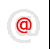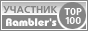Apache-Talk @lexa.ruInet-Admins @info.east.ruSecurity-alerts @yandex-team.runginx-ru @sysoev.ruđéűéôĺđéóříááňčé÷ :: Filmscanners
Filmscanners mailing list archive (filmscanners@halftone.co.uk)

### [filmscanners] RE: Density vs Dynamic range

```Hi Julian,

> This HAS to be a problem of semantics!

I'm beginning to believe that...

> You are waging a war on so many

I don't consider it war at all!

> You say:
> "Simply put, dynamic range is the (max value - min value) divided
> by noise."

Correct...when expressed in simple values...

> But NO - dynamic range is max value - min value (when expressed as a
> difference) ***OR*** max value divided by min value (as a ratio).

Hum.  I don't recall saying that...BUT...it IS max - min, when min is NOISE
AND they both are in log values!

> Since we all agree that min value = noise level,

Ah, but that's not always the case, and I've never made that
assumption...BUT...the ISO proposal does, and I'm not sure I agree with that
assumption...

> then you are including
> noise level twice in the equation/definition and thus confusing things
> immeasurably.

Well, it shouldn't confuse things...but as I said, I don't make the
assumption that min value is noise.  It certainly CAN be noise...

>  You say that
>
> DR = (max - min) / noise

Correct, and I, short hand, don't include the log etc. since it's obvious
(at least to me) when log applies and when it doesn't...and log is simply
another way of nomenclating something, so it matters not to me whether it's
used or not, the concepts are still the same.

>          = (max - noise) / noise

If...min value is equal to noise, then yes...

> But this is just not true. And I know that you know it is not true because
> you say so at other times, and your references say so, which is
> why I think
> we have a semantic problem here.

Hum.  I'll see where you're going with this...but re-read what I said above
if you're convinced that is what I've said...because the above contradicts
that, and I don't believe I've said what you believe I've said...

> You say:
> >the Dynamic Range equation out of "Digital Signal Processing in VLSI":
> >DR (dB) = 10log10(largest signal/smallest discernable signal) or:
> >3.6 is equal to 10**3.6 or 3981, and .2 is 10**.2 or 1.5849...
> >SO...
> >DR (in Bells, not DECI-Bells) = log10(3981/1.5849)
> >Which, is 3.4 ;-)
>
> This is correct.  There it is - you say DR = max/min which is the ratio
> expression of dynamic range.  NOT (max-min)/min.  Just max/min.

They are the same thing, it's all in what your definition of max etc. is.
Note in my provided diagram, "largest" is the range...and is actually (max
value - min value).  But, you instead of saying "largest" (which I don't
like as the term, BTW, I prefer the term "absolute range" or something a bit
more descriptive of what is actually meant).

Any range has a top and a bottom, and the absolute range is top - bottom.
If you are measuring from 1 volt to 3 volts, you have a 2 volt range.  You
can either express it as (3 - 1) or 2.

> That is
> the ratio version of the definition of DR.

The ratio is used simply for non-log values...

> Dynamic range is a
> range, not a
> number of steps, by definition.

No, it is not.  It is a DYNAMIC range.  Range has a meaning all in and of it
self.  If it was a simple range, as you suggest, it would simply be called a
range...but it's called "dynamic range".

>From another one of my posts:

Look up the word dynamic:

"Characterized by continuous change, activity"

In other words, the changes over a given range...and change must be
perceived for it to be change.  You must specify the range for dynamic range
to be meaningful.  Obviously, if you double the range you are resolving over
(and keep the resolution the same), you get a higher dynamic range (and a
higher range)...but no better resolution.

> It happens that the OPTIMUM number of
> steps is equal to the number that is the DR expressed as a ratio to
> one.  But this is not the same as saying that the DR is defined as the
> number of steps.  It is not, it is simply a RANGE, usually expressed as a
> ratio.

And this I completely disagree with.  Dynamic range defines the resolution
within a range, and a "range" simply specifies the endpoints, and says
nothing about the resolution within that range.

Here's an easy example.  You measure one foot in 1" increments.  That gives
you a min of 1 and a max of 12.  Dynamic range = max/min, or 12 in this
case.  Range is still 12.  Now change your increment to 1/4".  Dynamic range
= min/max, or 12/.25 and the result is 48.  Hum.  Over the EXACT same range,
we now have two different DYNAMIC ranges.

> Simple example.  Signal with 3mW of noise and 30mW max.  The dynamic range
> from first principles is simply "3 to 30mW".  There it is -  as a real
> basic range.  "3 to 30mW".

Dynamic range is NEVER specified like that.  Your example is incorrect.

> This can be expressed as a single figure in two ways - as a
> ***difference*** or as a ***ratio***.  As a difference, the DR is
> 27mW,

Yeah, but that isn't the result of ANY dynamic range equation.

> but
> this is difficult to use because you have to know the upper or
> lower limit,
> so it is really "27mW with a top of 30mW" or "27 mW with a bottom of 3mW".

I don't understand how that's meaningful...

> For this reason and because the range is so great normally, obviously we
> usually express it as a ratio.  In this case the DR as a ratio is 30/3 or
> 10.  The Dynamic range is 10, this number expresses a RANGE as a ratio
> between largest and smallest. You can dB or B it if you like.

You are assuming that 3 is the noise level, and if it is, your equation is
correct.

> So, again, the dynamic range is ***either***    MAX -
> MIN  ***OR  ***  MAX/MIN  depending on which is most useful to you.

Wrong.  Max-min is used for LOG values.  Max/Min is used for non-log values.

> The
> standard in signal theory as you know is as you quoted it above - MAX/MIN,
> or at least the log form of that.

Er, and the log form of that is max-min...

> But it is NOT  ...      (MAX - MIN) / MIN which is what you keep
> saying and
> is at the core of the problem here.

Yeah, but it's right...and is right out of the book!

> The difference between the two points of view is twofold,

It's not two points of view...they are all the same thing, if you understand
WHY they are the same thing.

> one is this
> "equation difference" and the other is the definitional difference where
> you keep equivalencing "number of steps" with "dynamic range" just because
> the OPTIMUM number of steps happens to have the same number as the dynamic
> range when expressed as a ratio to one.

It's not "just because", dynamic range IS ABSOLUTELY the same as the "number
of steps" or "number of discernable values".  It's just what dynamic range
is.  Part and parcel of the concept!

> Dynamic range is dB (usually), while number of steps is n, a number, not a
> ratio.

Er, dynamic range, in dB is NOT a ratio either.  It is a simple number, just
a log number.  If dynamic range is 78dB, that means there are 10**7.8
differentiable levels.

> You get the OPTIMUM number of steps n from the dB ratio, but they
> are NOT the same thing, despite being the same actual number when
> expressed
> in one particular way.

I'm not clear what you mean here...

> As for definition of dynamic, here is where I really do not agree with
> you.  Maybe radar and hi-fi and other areas are different from scanners,
> but I don't think so.

No they don't, and all the statements I've made here, are the exact same
with audio (which I've designed a lot of digital audio gear, and written the
specs for it), and I've done minimal radar.  In audio, the DR equation is
20log10((max-min)/noise)*(1.5)...so it's a bit different equation, but
conceptually exactly the same.

> Lastly,
>
> Julian R. said:
> > > So the optimum number of bits is decided by:
> > >
> > > num coded levels =  max level divided by optimum digital step size
> > >                  = max level divided by noise level.
> Austin replied:
> >Er, that is EXACTLY THE SAME FRIGGING EQUATION I USED.  GRRRR!!!!!
> >...But yet here, you are DIVIDING IT BY NOISE.  What am I missing here?
>
> What you are missing in terms of what I am trying to say is <<< what is on
> the left of the equals sign>>> (POINT 1).  There is also one term
> different
> in the equations we use (POINT 2).
>
> POINT 1:  You would put dynamic range on the left of the equals sign and I
> would not.  I put "optimum number of levels".  As we keep realising, the
> optimum number of levels EQUALS the dynamic range expressed as a ratio to
> one, but that is not saying they are the same thing.

I'll skip this point for now, as I believe other things I've said clarify my
position on this...

> POINT 2: You in fact do say this at times (I.e. max level divided by noise
> level), but at other times you say  (max level - min level) divided by
> noise level.  These are different, not so much in the number that results
> because the variation is usually small, but in terms of the MEANING and
> DEFINITION that it implies.

Again, largest is equal to the "top" - "bottom" of the signal.  As I said
above, a range of 1V to 3V is equal to 2V, and you can either say (3 - 1) or
2, they, obviously, are interchangeable...and are obviously EXACTLY the
same.

> Are we converging?

immediately assume it's wrong...please assume it's right, and see what it is
saying.

Regards,

Austin

----------------------------------------------------------------------------------------
Unsubscribe by mail to listserver@halftone.co.uk, with 'unsubscribe
filmscanners'
or 'unsubscribe filmscanners_digest' (as appropriate) in the message title or
body

```Copyright © Lexa Software, 1996-2009.